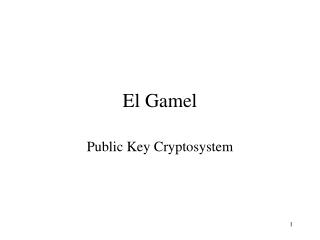DownloadDownload PresentationEl Gamel

# El Gamel

Télécharger la présentation## El Gamel

- - - - - - - - - - - - - - - - - - - - - - - - - - - E N D - - - - - - - - - - - - - - - - - - - - - - - - - - -
##### Presentation Transcript

1. El Gamel Public Key Cryptosystem

2. The Discrete Log Problem The El Gamel public key cryptosystem is based upon the difficulty of solving the discrete logarithm problem (DLP) which is as follows: Given a prime p and values g and y, find x such that y = gx mod p

3. For a small value of p, it is easy to solve a DLP by trial and error or exhaustive search. • For example, given p = 11, g = 2 and y = 9, we can try different values of x until we reach the correct solution for 2x mod 11 = 9 • However, for a large value of p, i.e., if p has around 100 decimal digits, then it is not possible to solve a DLP using current technology.

4. El Gamel • El Gamel is a public key cryptosystem with security which relies on the difficulty of solving a discrete log problem. • If you can solve the DLP then you can crack El Gamel.

5. El Gamel Key Generation Bob generates public and private keys as follows: • He picks a large random prime p • He finds a generator g mod p (i.e. gx mod p gives a different answer for every value of x, which means that gp-1 mod p is the first time the answer is 1).

6. He picks a random number a between 1 and p-1. • He computes y = ga mod p The public key is (p, g, y) The private key is a

7. El Gamel Encryption If Alice wants to send Bob a message, she looks up Bob’s public key (p, g, y) and breaks the message up into blocks with each block less than p. Then for each message block m she takes the following steps:

8. She generates a random number k between 1 and p-1. • She computes r = gk mod p x = yk mod p c = (m * x) mod p • She sends Bob the values r and c.

9. El Gamel Decryption Bob receives the ciphertext (r,c) from Alice. He decrypts it as follows: • He computes ra mod p = x {ra = (gk)a = gka = gak = (ga)k = yk = x} • Now he can solve c = (m * x) mod p to find the value of m. Only Bob can do this because only Bob knows the value of the private key a.

10. RSA Security based on the difficulty of the factorisation problem. The ciphertext is just one value c which is roughly the same size as the message m. El Gamel Security based on the difficulty of the discrete log problem. The ciphertext is two values c and r and so is twice the size of the message m. Comparing RSA and El Gamel

11. RSA The encryption and decryption algorithms are the same (modular exponentiation). RSA is a patented algorithm. El Gamel The encryption and decryption algorithms are different (although both take about the same time to perform). El Gamel has no patent. This gives it a financial advantage over RSA.

12. The Elliptic Curve DLP • A further advantage of the El Gamel cryptosystem, is that it can be generalised to a cryptosystem based on the discrete log problem for elliptic curves. • This appears to be even harder to solve which means that the prime used can be smaller and so encryption is faster.

13. Applications of public key cryptosystems. Public key cryptosystems are generally less efficient but more secure than symmetric key cryptosystems. In practise public key systems are used for • Digital signatures • Key exchange

14. Digital Signatures • A digital signature for a message from a particular sender is a cryptographic value which depends upon both the message and the sender. • A digital signature provides data integrity (proof that the message hasn’t been altered) and non-repudiation (proof of origin - the sender cannot deny sending the message).

15. To digitally sign a message m using a public key cryptosystem, Bob simply encrypts the message m using his private key to get a signature s. • Bob then sends Alice both the message and the signature (m,s). • Alice decrypts the signature using Bob’s public key. If the decrypted signature is the same as the message m then Alice accepts the message is genuine and from Bob.

16. Digital Signature using RSA • Bob takes a message m and uses his private key d to compute the signature s = md mod n 2.Bob sends Alice the pair (m,s) • Alice decrypts the signature s using Bob’s public key e to compute m1 = se mod n • If m1= m then Alice accepts the message as genuine because only Bob knows the private key d which works in conjunction with the public key (n,e).

17. DSS Recall that in the El Gamel public key cryptosystem, the algorithms for encryption and decryption are not the same. This means that using El Gamel for digital signatures is not as straight forward as using RSA. However, the Digital Signature Standard (DSS) is an alternative signature scheme to RSA which is based upon El Gamel.